## Cleaning Datastream downloadsUsing ISIN codes is very often a good choice when you download data from Datastream and want to combine it with other data. ISIN codes are also regularly available in other databases. For the Datastream downloads you will have to seperate the ISIN again from the code of the variable which (as a default) is presented after the ISIN code between brackets.

Because every ISIN code consists of 12 digits, seperating them in Excel is easy: use the function =left(). Example: =left(CELL; 12)In Stata you can use the substring command substr() to do something similar.
Example: gen isin=substr(Code, 1, 12)Seperating the code of a company from the code of the variable in Datastream is more difficult if the company codes have varying lengths. The Datastream code (Datastream mnemonic) for instance, is such a code. If you need to seperate this from the code of the variable you need to make use of the length of the variable code which remains the same. To do this we combine to Excel functions: =left() and =len(). Example: =left(Cell; len(Cell)-11)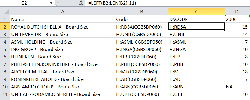In Stata a similar thing can be done using a combination of substr() and strlen(). Example:
gen dscd=substr(variable, 1 ,(strlen(variable)-11))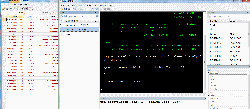Another thing which you may find necessary is seperating the names of the variables from the names of the companies. The variable name is most easily seperated since the name is a fixed number of characters. Again similar functions using the length of the content of CELLS can be used. A different way of seperating both is using a combination of the Left() function combined with the function Find(). For most downloads from Datastream both items are combined but there is a common element seperating the two, namely: ” “.
Using this we can build the following formula: =left(CELL;(FIND(” – “;CELL)))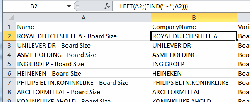In Stata a similar option is available to split a combined variable. The function split can be used as follows: split Name, generate(deel) parse(” – “). This generates two new variables that both start with the name deel and are numbered, for example: deel1 deel2. Afterwards you rename the variables deel1 and deel2 to what you need.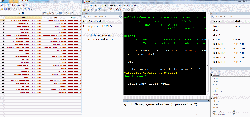Email

## Excel and Filling up blank cellsRecently I got a question on how to arrange the data in Excel for an analysis where some data was missing. Below here you see an example download from the database Joint Ventures & Alliances from SDC Platinum. Not every row has data but each deal starts on a new row with the date variable “Alliance Date Announced”. Each alliance also has a unique Deal number (3rd column):In some programs (to do an analysis of the data) what you need is to have the data repeated for each row (observation) related to that specific instance and not any rows with blanks (unless a variable for a row/observation contains no data of course). In the following example the data looks like the previous picture, incomplete: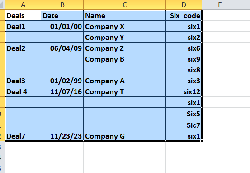You want it to look as follows: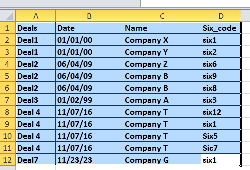Excel has an option that allows you to automatically fill in all the blanks. This works as follows:

Step 1: Make sure that you click on a cell inside the data table

Step 2: In Excel click the option in the Excel ribbon: Find & Select > Go To Special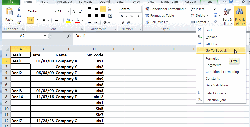Step 3: In the pop-up mark the option: Blanks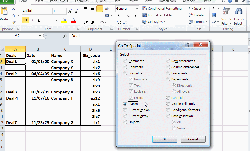Step 4: You see that Excel now tries to find all the empty cells in the active table and they are highlighted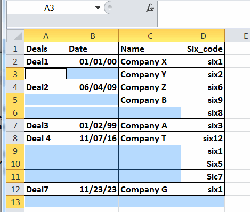Step 5: Use your keyboard to type “=
Step 6: Now click the Upwards arrow on your keyboard
Step 7: Use the keycombination: CTRL + Enter

N.B. 1: Please remember that after the final row Excel tends to add another row. In this case you need to delete it again as this would only add a duplicate observation to the table.
N.B. 2: Dates may be filled in as numbers. This is fine because in Excel dates are really numbers (number 1 is 1-1-1900). Just use formatting to make sure these numbers are presented as dates again

Warning: When you use the option to complete data for each observation, be sure that the correct data is duplicated. In the example output from SDC Platinum (below) you see columns that relate to events but which are spread over more rows. It may be an idea to copy parts of the data to separate sheets (for instance using unique event/deal numbers) to duplicate data there and later merge the data tables again. See columns G, I and J.Email

## Excel and duplicates in a datasetIn the past I posted items on duplicate data from datasets (from Compustat databases) and how to find this out using programs like stata. For smaller datasets the program Microsoft Excel can also be used to investigate your datasets. AT some point, when merging datasets from multiple sources it may happen that you get duplicate data. Using Excel functions like a pivot table (draaitabel) and vlookup (vertikaal zoeken) duplicate data can be detected as follows:

1) first you need to tell Excel that your dataset is a specific delineated table using the menu option: Insert > Table2) When you insert this option you need to indicate the range and if there are any column headers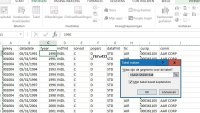3) The same menu tab insert (invoegen) has the option to create a summary pivot table (samenvatten met draaitabel). Select this option at the top left corner: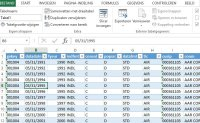4) Create the PivotTable in a new (or empty) sheet:5) The empty Pivot Table will be shown as follows: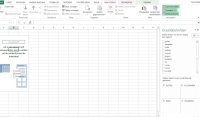6) In this example I drag the fields I want to check for duplicates (records/observaions) down to rows (Rijen). At the box values I drag a random field (in this case indfmt). On the left side of the screen the result will be presented: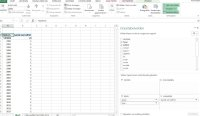7) In this example I click the field gvkey and choose the option to change the field settings8) At subtotals & filters mark the second option No(ne)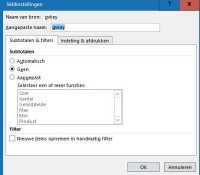9) In the tab Format & Print (Indeling & Afdrukken) mark the option: item labels in table format (Itemlabels in tabelvorm weergeven). Also make sure to mark the option to repeat item labels (Itemlabels herhalen):10) The result chould look as follows: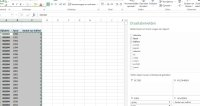11) Copy the list to a new sheet and use the concatenate function (Tekst.samenvoegen) to create a combination of GVKEY and year:12) Create a similar link list with the Concatenate function in the original Compustat datasheet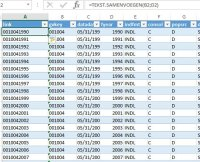13) Now use the VLOOKUP function in Excel in the Compustat datasheet to look up the link in the sheet with the result of the Pivottable. Make sure that the VLOOKUP option says False (Onwaar) at the 4th option as follows:14) Finally, using the filter option you can find out if there are duplicatesd by selecting everything for which the count is higher than 1. The tricky thing will be deciding what to do with the result.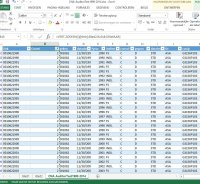N.B.: If a dataset has over 100.000 observations the process described above will take some time as Microsoft Excel will require significant processing power from the computer. For larger datasets I reccomend using Stata.Email

## Thomson Eikon & Event windowsNot too long ago I was working with the successor of Thomson 1 / Thomson One which is called Eikon or Thomson Eikon. This product is web-based and is similar in look and style as Bloomberg. I found out that in most cases for me the Excel add-in was the most useful part and allowed me to download larger amounts of data.
The specific Add-in did, however, miss a key feature which I use heavily in Datastream: there is no Request Table tool to allow event studies with changing dates and time windows. Using the add-in I was able to figure out how to still do something like this using the commands which Thomson inputs in your Excel worksheet to call up the data.

With a bit of help from the Eikon Helpdesk I was able to come up with a template that kind of does the same thing as a Request Table Excel tool from Datastream. You can download the example here.

Explanation of the worksheet:

0) Before using the worksheet template, make sure that the connection to Eikon on your computer is not yet live. Otherwise the sheet will immediately start updating as the Add-in and Eikon code is usually set up this way.

1) The first sheet is called Events
As the name already suggests, this is where you put the codes for the series (column A). In columns B and C the Start and End dates for the series go. If you need an exact number of trading days (of data) you can use the formulas from a previous post.
If the exact number of days need not be the exact same column D shows the number of calendar days between the start and end days. This is done by deducting the end date from the start date. This works fine as dates are also numbers in Excel. Cell E2 shows the highest number in the entire column D using formula =MAX(D:D)2) The second sheet is Data
The example sheet is used to download the Eikon data into and is based on generated Eikon code(s).

Cell D1 in sheet Data is linked to Cell E2 from the Events sheet and will show the same value with the formula: =Events!D2
This number is important as this indicates the (maximum) number of rows for each new window to start downloading data without overwriting the data from previous (downloaded) series. This may cause empty rows between series/data, but these empty rows can be deleted afterwards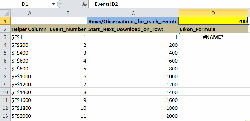The formulas in Column C are used to calculate the row/cell numbers for each separate series to start downloading: =((B4-\$B\$3)*\$D\$1)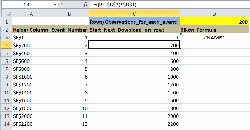This is also based on the generated number of events in columb B: =B3+1.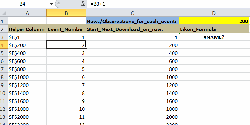The Helper Column is column A. This column is needed to get the download destinations to be included later in the Cells with the Thomson Eikon codes. There is just a technical reason for this, otherwise the Eikon code does not accept the generated destination cells. The formula is: =ADDRESS(\$C4;6) where the \$C4 is the number of rows it takes from column C and 6 indicates the column number where the download should start.The download code from eikon is as follows: =TR(Events!\$A2;”TR.PriceClose”;”Frq=D SDate=#1 EDate=#2 Curn=EUR CH=Fd;date;IN Transpose=Y NULL=NA CODE=ISIN”;INDIRECT(\$A3);Events!\$B2;Events!\$C2)• This formula takes the series data from the Events tab in column A where it says Events!\$A2
• The start & end dates are included at the end where it says: Events!\$B2;Events!\$C2
• The data download cell destinations for the events are indicated where it says: INDIRECT(\$A3)

You just copy everything downwards (including the Eikon formula) depending on the number of events in the dataset.

3) When you are done copying the events in to the sheet Events and copying the necessary formulas in the Data sheet, you can then go live by signing in into Eikon. You will see that the data download starts automatically.

N.B.: The example download sheet downloads the closing price for series with the Eikon datatype code TR.PriceClose. If you need another datatype this part needs to be changed in the formula.Email

## Working with Compustat Execucomp tenure dataNot too long ago I had a question from someone who was having trouble working with data from Compustat Execucomp. He wanted the yearly tenure for a specific group of people with the function of Chief Financial Officer. The research spanned a period of 2009-2014 (post-crisis). The data that was downloaded looked something like this: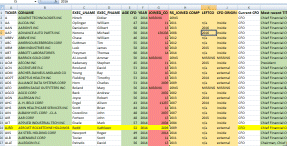Step 1: Data cleaning
One of the first steps to take in this case is to make sure to have the right kind of data to work with. In this case the columns H and I needed to be checked and cleaned. In column I you see the date when a person left as CFO working for the company. In this situation we see items like n/a where the data is unavailable and this means that the person still continues to work as CFO for the company. We first need to replace such values with the value 2014 for the last year of our research as we are looking for the tenure within the time frame 2009-2014. Any other years after 2014 can in this case also be replaced with 2014. You can use the search and replace function in Excel to do this step by step. Afterwards you can use the Filter option in Excel to check for weird data or outliers. In principle you have to check both columns with start year and left year to be sure there are no outliers (weird values).In column H you see the year when a person joined the company. I am assuming that this was also the startyear for each person when he came to work as CFO at the company (I have not personally checked this). You see in the screenshot that not every year is seen as a numerical value: Excel shows little green triangle dots in the cells where it thinks the data is text. To ensure that a year is seen as a numerical value you can add a new column and use a trick to create numerical values in this column: devide cell by 1. See screenshot column J for the original data and column K for the new years. In the top left corner you see the “formula” you can copy downwards for all years.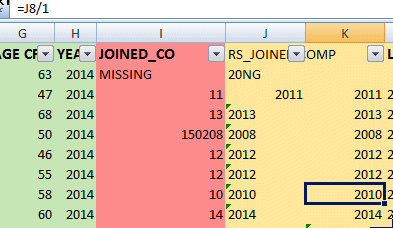Step 2: Calculate tenure for each year
In the example for this blog I only calculated the tenure for the final years of the research time frame (2013 and 2014). You can figure out the formulas for the other years. First I started calculating the CFO tenure for 2014. In this example I assume that if the startyear matches the lastyear someone has worked in this capacity for less then a full year making the tenure less then 1 and thus zero. In this case I get the right number of years of the tenure by substracting the startyear from the lastyear (= research year 2014). See example: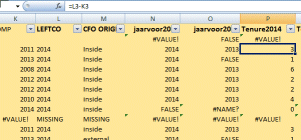Now for the tenure of the previous year (2013) the If statement comes in handy to figure out the tenure for this year. The full formula is:
=IF(K3<=(L3-1);(P3-1);FALSE)

K3 = start year tenure within the research window (or before)
L3 = last year for the research window (2014)
P3 = tenure for the final year of the research window (= 2014)

The formula in essence does the following: if the previous year (in this case 2013) matches the start year (or is smaller), then the tenure is that of 2014 minus 1. If not then put the word False there. This last condition prevents outliers from causing problems. Screenshot: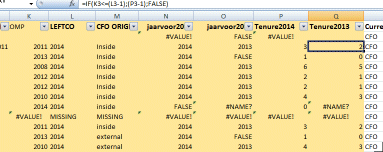The same formula can also be used for the previous years. All you need to do is change the formula for the right numbers. 2012 example: =IF(K3<=(L3-2);(P3-2);FALSE)

Step 3: Figure out the relevant years
This step is essentially not necessary as the filter option of excel is already available to make an annual selection by year but you then have to add the tenure year manually for each year after copying the relevant tenure data by year (to a new sheet).
The formulas in step two will provide a tenure of  0 (or more) as long as the end year for the tenure (within the time frame 2009-2014) is equal to (or higher) then the start year for the tenure (within the time frame or earlier). To know the tenure by year we create columns to show which tenure applies to what year. That allows us to use a filter in Excel to more easily get the relevant data where there is a tenure of more then zero. I created the columns N and O to get the tenure years for 2014 and 2013. The formula I used for 2014 is: =IF((P4>0);RIGHT(\$N\$1;4);FALSE)
where: P4 = calculated number of tenure years for 2014. I have put the year in the name in the first cell at the top of the column (first) as the last 4 digits making it possible to use \$N\$1.
Example: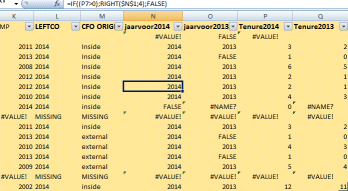For 2013 all you need to change is the cell P4 into Q4 and the header \$N\$1 into \$O\$1. You also need to put the year in the name of the variable at the top of the column). Subsequent years work the same way.

Step 4: Filter the data for the relevant years
As the final step you can now use the standard filter option to copy the relevant data by year to a new sheet.I would then also remove irrelevant data for other years which do not apply to the specific year I have filtered for. The end result would look something like this: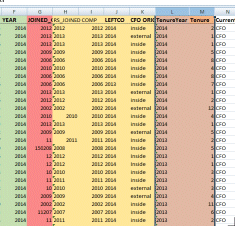Email

## Abnormal Return & market choiceToday I had a student who wanted the abnormal return for a couple of lists of stocks but wanted to choose different markets depending on the stock. To get the Abnormal Return from datastream you can use the following formula:

REGR#(PCH#(X(LI),1D),PCH#(X*1.0000000,1D))

I posted about the formula earlier and the post explains that the LI stands for the comparison Local Market Index. In this case that is not the index that was needed for some of the lists that the student wanted to use, because, LI usually is the index of the main exchange where a company is listed. See the previous post on how to find out which index for each equity this is.

To change the formula, you can exchange the market by replacing the X(LI) with a Datastream mnemnonic for an index. Example for the Dutch midkap index AMSMKAP:

REGR#(PCH#(AMSMKAP,1D),PCH#(X*1.0000000,1D))

If you also need to change to multiple indices/markets for a list the same method can be used to generate formulas like I did for generating a list of BETA formulas. You can download an example here for a list of Abnormal Return formulas. Using such a list an example Request table sheet in Datastream would look like this: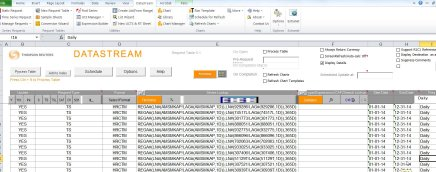The same thing also applies to other Datastream formulas, like the Alpha formula:

REGA#(LN#(X(LI)/LAG#(X(LI),1M)),LN#(X/LAG#(X,1M)),60M)

You can download an example Excel sheet here as well.Email

## Restoring unique codesIn the past I blogged about the fact that if you work with codes like SEDOL (or any other types), to get information on listed companies, it is important to keep them in tact. Excel, for instance, has a habit of removing zeros when they are the first digits of a code.
Some databases (like SDC Platinum) will not give you a good search result if codes are incomplete when you search using a list of these abbreviated codes. Some databases are more forgiving when you search on codes and the zeros in front are gone.
The second, more important reason, however, to keep the codes in tact (including zero digits in front) is, that when you combine datasets, you will probably use codes to match them. Example: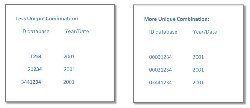For whatever reason, when you use Excel, you may have inadvertently have codes in a dataset that are no longer in tact. In the earlier blog post I solved this problem using a nested IF() function for Sedol codes. This example could be adapted for longer codes.
Recently, a student of the Vrije Universiteit, pointed out a more elegant solution: using the REPT() and LEN() functions. The advantage of this solution is, that it can very easily be adapted for codes of a different length. The solution function is:

=REPT(“0”;X-LEN(Cell))&Cell

Explanation: The function will repeat/add the character Zero (= 0) to the characters in a Cell and the number of zeroes depends on the X minus the Length (= len() ) of the number of characters already in the Cell. Added to this will be the characters of the original Cell.

Example for Sedol codes (always 7 digits long):

If the abbreviated Sedol code is: 68G78
The Function would be (Sedol code is 7 digits long): = REPT(“0”;7-LEN(Cell))&Cell
The result would be: 0068G78

Below you see an example for abbreviated 9-digit CUSIP codes: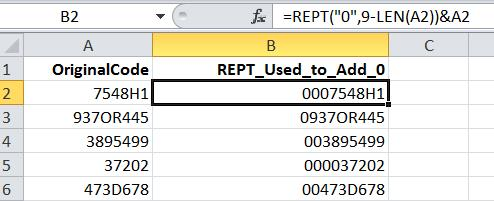Email